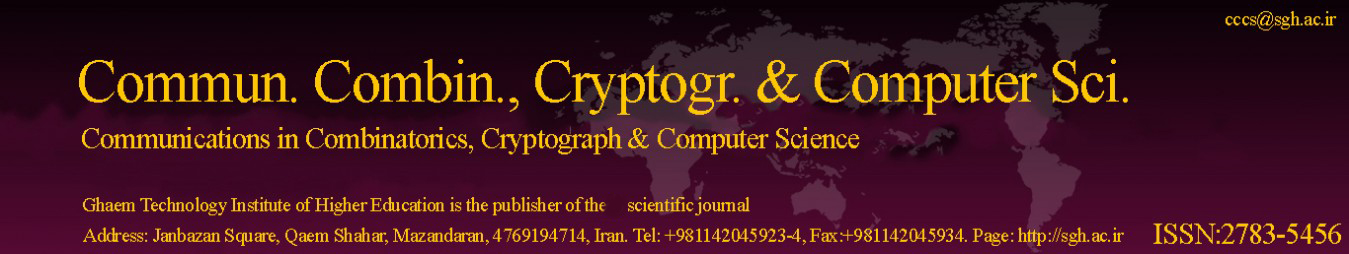Original Research Article

Article volume = 2021 and issue = 1

Pages: 44–49

Article publication Date: November, 1, 2021

# Lee Weight for Direct Sum of Codes

#### Farzaneh Farhang Baftani

Department of Mathematics, Ardabil Branch, Islamic Azad University, Ardabil, Iran.

##### Abstract:

Let C be a linear code of length n over Z4. The Lee support weight of C, denoted by wtL(C), is the sum of Lee weights of all columns of A(C) that A(C) is the jCj n array of all code words in C. For 1 6 r 6 rank(C), the r-th generalized Lee weight with respect to rank (GLWR) for C, denoted by dLr (C), is defined the minimum of all Lee weights of Z4-submodules of C with rank = r. In other words dLr (C) = minfwtL(D);D is a Z4 - submodule of C with rank(D) = rg For linear codes C1 and C2 over Z4 of length n1 and n2, respectively, the Direct Sum of them ,denoted by C1 C2 , is defined as follows: C1 C2 = f(c1, c2) : c1 2 C1, c2 2 C2g. Motivated by finding dLr (C1 C2) in terms of dLr (C1) and dLr (C2), we investigated dLr (C1 C2) and we obtained dLr (C1 C2) for r = 1, 2. Moreover, we generally obtained an upper bound for dLr (C1 C2) for all r, 1 6 r 6 rank(C1 C2).

##### Keywords:

Linear code, Hamming Weight, Lee Weight, Generalized Lee Weight, Direct Sum of Codes.

##### References:
•  S.T. Dougherty, M. K. Gupta, K. Shiromoto, On Generalized Weights for codes over Zk, Australian Journal of Combinatorics., 31(2005), 231–248.
•  S. T. Dougherty and S. Han, Higher Weights and Generalized MDS Codes, Korean Math. Soc., No. 6(2010),1167–1182.
•  S.T. Dougherty, S. Han and H. Liu, Higher weights for codes over Rings,Springer - Verlag, (2011). 1, 2.2
•  F. Farhang Baftani and H. R. Maimani, The Weight Hierarchy of Hadamard Codes, Facta UniverSitatis (NIS), 34(2019), No. 4, 797—803. 1
•  F. Farhang Baftani and H. R. Maimani, The Weight Hierarchy of Ham(r, q), Italian Journal of Pure and applied Mathematics, 45( 2021). 1, 2.2
•  G. L. Feig, K. K. Tzeng and V. K. Wei, On the Generalized Hamming Weights of Several Classes of Cyclic Codes, IEEE Trans. Inform. Theory, 38(1992), No. 3, 1125-1126. 1
•  B. Hove, Generalized Lee Weight for Codes over Z4, Proc. IEEE Int. Symp.Inf. Theory, Ulm, Germany (1997),203. 1 1
•  J. H. Van Lint, Introduction to Coding Theory, Springer- Verlag, (1999). 1 1
•  V.K.Wei, Generalized Hamming Weights for linear codes, IEEE Trans. Inform. Theory 37(1991) ,no.5,1412–1418. 1
•  B.Yildiz, Z. Odemis Ozger, A Generalization of the Lee Weight to Zpk, TWMS J. App. Eng. Math. 2(2012), no.2, pp 145–153. 1